pdemesh

Description

example

pdemesh(model) plots the mesh contained in a 2-D or 3-D model object of type PDEModel.

example

pdemesh(mesh) plots the mesh defined as a Mesh property of a 2-D or 3-D model object of type PDEModel.

example

pdemesh(nodes,elements) plots the mesh defined by nodes and elements.

example

pdemesh(model,u) plots solution data u as a 3-D plot. This syntax is valid only for 2-D geometry.

example

pdemesh(___,Name,Value) plots the mesh or solution data using any of the arguments in the previous syntaxes and one or more Name,Value pair arguments.

example

pdemesh(p,e,t) plots the mesh specified by the mesh data p,e,t.

example

pdemesh(p,e,t,u) plots PDE node or triangle data u using a mesh plot. The function plots the node data if u is a column vector , and triangle data if u is a row vector.

If you want to have more control over your mesh plot, use pdeplot or pdeplot3D instead of pdemesh.

h = pdemesh(___) returns handles to the graphics, using any of the arguments of the previous syntaxes.

Examples

collapse all

Create a mesh plot and display the node and element labels of the mesh.

Create a PDE model. Include the geometry of the built-in function lshapeg. Mesh the geometry.

model = createpde;
geometryFromEdges(model,@lshapeg);
mesh = generateMesh(model);

Plot the mesh.

pdemesh(model)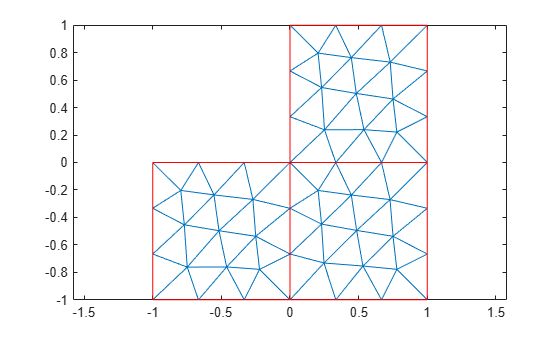Alternatively, you can plot a mesh by using mesh as an input argument.

pdemesh(mesh)Another approach is to use the nodes and elements of the mesh as input arguments for pdemesh.

pdemesh(mesh.Nodes,mesh.Elements)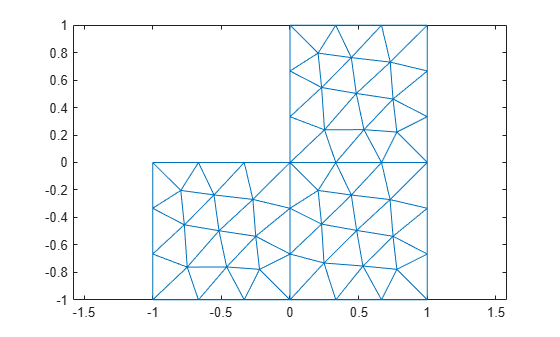Display node labels.

pdemesh(model,'NodeLabels','on')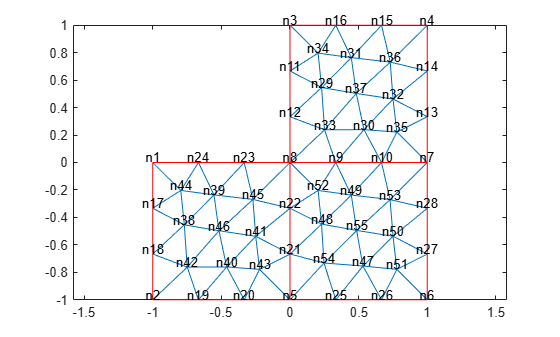Use xlim and ylim to zoom in on particular nodes.

xlim([-0.4,0.4])
ylim([-0.4,0.4])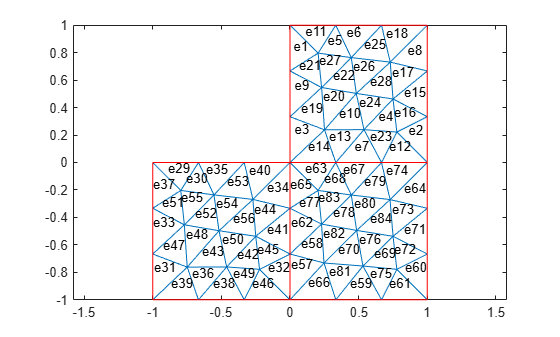Display element labels.

pdemesh(model,'ElementLabels','on')
xlim([-0.4,0.4])
ylim([-0.4,0.4])Apply boundary conditions, specify coefficients, and solve the PDE.

applyBoundaryCondition(model,'dirichlet', ...
'Edge',1:model.Geometry.NumEdges, ...
'u',0);
specifyCoefficients(model,'m',0,...
'd',0,...
'c',1,...
'a',0,...
'f',1);
generateMesh(model);
results = solvepde(model)
results =
StationaryResults with properties:

NodalSolution: [1177x1 double]
Mesh: [1x1 FEMesh]

u = results.NodalSolution;

Plot the solution at nodal locations by using pdemesh.

pdemesh(model,u)The pdemesh function ignores NodeLabels and ElementLabels when you plot solution data as a 3-D plot.

Create a PDE model, include the geometry and mesh it.

model = createpde;
importGeometry(model,'Plate10x10x1.stl');
generateMesh(model,'Hmax',5);

Plot the mesh setting the transparency to 0.5.

pdemesh(model,'FaceAlpha',0.5)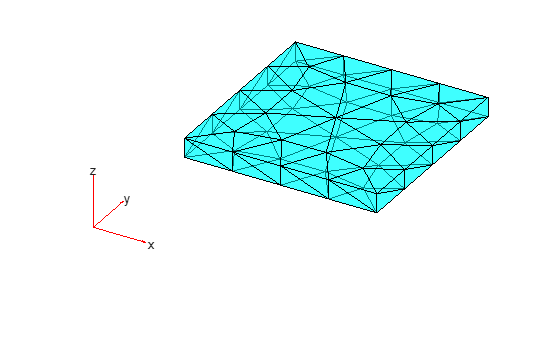Find the elements associated with a geometric region.

Create a PDE model.

model = createpde;

Include the geometry of the built-in function lshapeg. Plot the geometry.

geometryFromEdges(model,@lshapeg);
pdegplot(model,'FaceLabels','on','EdgeLabels','on')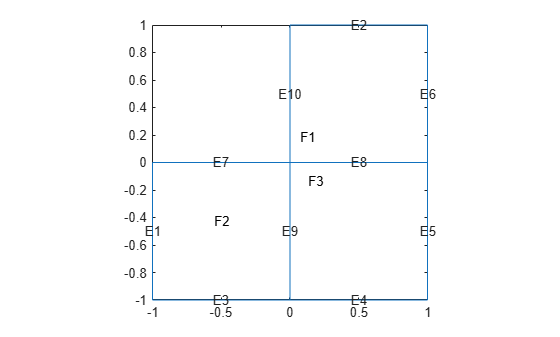Generate a mesh.

mesh = generateMesh(model,'Hmax',0.5);

Find the elements associated with face 2.

Ef2 = findElements(mesh,'region','Face',2);

Highlight these elements in green on the mesh plot.

figure
pdemesh(mesh,'ElementLabels','on')
hold on
pdemesh(mesh.Nodes,mesh.Elements(:,Ef2),'EdgeColor','green')Plot the mesh for the geometry of the L-shaped membrane.

[p,e,t] = initmesh('lshapeg');
[p,e,t] = refinemesh('lshapeg',p,e,t);
pdemesh(p,e,t)Now solve Poisson's equation $-\Delta u=1$ over the geometry defined by the L-shaped membrane. Use Dirichlet boundary conditions $u=0$ on $\delta \Omega$, and plot the result.

u = assempde('lshapeb',p,e,t,1,0,1);
pdemesh(p,e,t,u)Input Arguments

collapse all

Model object, specified as a PDEModel object, ThermalModel object, StructuralModel object, or ElectromagneticModel object.

Example: model = createpde(3)

Example: structuralmodel = createpde('structural','static-solid')

Example: emagmodel = createpde('electromagnetic','electrostatic')

PDE solution, specified as a vector or matrix.

Example: results = solvepde(model); u = results.NodalSolution; or u = assempde(model,c,a,f);

Mesh object, specified as the Mesh property of a PDEModel object or as the output of generateMesh.

Example: model.Mesh

Nodal coordinates, specified as a 2-by-NumNodes matrix for a 2-D mesh and 3-by-NumNodes matrix for a 3-D mesh. NumNodes is the number of nodes.

Element connectivity matrix in terms of node IDs, specified as an NodesPerElem-by-NumElements matrix. NodesPerElem is the number of nodes per element. Linear meshes contain only corner nodes, so there are three nodes per a 2-D element and four nodes per a 3-D element. Quadratic meshes contain corner nodes and nodes in the middle of each edge of an element. For quadratic meshes, there are six nodes per a 2-D element and 10 nodes per a 3-D element.Mesh points, specified as a 2-by-Np matrix of points, where Np is the number of points in the mesh. For a description of the (p,e,t) matrices, see Mesh Data as [p,e,t] Triples.

Typically, you use the p, e, and t data exported from the PDE Modeler app, or generated by initmesh or refinemesh.

Example: [p,e,t] = initmesh(gd)

Data Types: double

Mesh edges, specified as a 7-by-Ne matrix of edges, where Ne is the number of edges in the mesh. For a description of the (p,e,t) matrices, see Mesh Data as [p,e,t] Triples.

Typically, you use the p, e, and t data exported from the PDE Modeler app, or generated by initmesh or refinemesh.

Example: [p,e,t] = initmesh(gd)

Data Types: double

Mesh triangles, specified as a 4-by-Nt matrix of triangles, where Nt is the number of triangles in the mesh. For a description of the (p,e,t) matrices, see Mesh Data as [p,e,t] Triples.

Typically, you use the p, e, and t data exported from the PDE Modeler app, or generated by initmesh or refinemesh.

Example: [p,e,t] = initmesh(gd)

Data Types: double

Name-Value Arguments

Specify optional comma-separated pairs of Name,Value arguments. Name is the argument name and Value is the corresponding value. Name must appear inside quotes. You can specify several name and value pair arguments in any order as Name1,Value1,...,NameN,ValueN.

Example: pdemesh(model,'NodeLabels','on')

Node labels, specified as the comma-separated pair consisting of 'NodeLabels' and 'off' or 'on'.

pdemesh ignores NodeLabels when you plot solution data as a 3-D plot.

Example: 'NodeLabels','on'

Data Types: char | string

Element labels, specified as the comma-separated pair consisting of 'ElementLabels' and 'off' or 'on'.

pdemesh ignores ElementLabels when you plot solution data as a 3-D plot.

Example: 'ElementLabels','on'

Data Types: char | string

Surface transparency for 3-D geometry, specified as the comma-separated pair consisting of 'FaceAlpha' and a real number from 0 through 1. The default value 1 indicates no transparency. The value 0 indicates complete transparency.

Example: 'FaceAlpha',0.5

Data Types: double

Color of mesh edges, specified as a short or long color name or an RGB triplet. By default, for 2-D meshes the edges within one face are blue (RGB triplet [0 0 1]) and the edges between faces are red (RGB triplet [1 0 0]). For 3-D meshes, the default edge color is black (RGB triplet [0 0 0]).

The short names and long names are character vectors that specify one of eight predefined colors. The RGB triplet is a three-element row vector whose elements specify the intensities of the red, green, and blue components of the color; the intensities must be in the range [0 1]. The following table lists the predefined colors and their RGB triplet equivalents.

RGB Triplet

Short Name

Long Name

[1 1 0]

y

yellow

[1 0 1]

m

magenta

[0 1 1]

c

cyan

[1 0 0]

r

red

[0 1 0]

g

green

[0 0 1]

b

blue

[1 1 1]

w

white

[0 0 0]

k

black

Example: 'EdgeColor','green'

Data Types: double | char | string

Color of mesh faces for 3-D meshes, specified as a short or long color name or an RGB triplet. The default face color is cyan (RGB triplet [0 1 1]). For details about available colors, see EdgeColor.

Example: 'FaceColor','green'

Data Types: double | char | string

Output Arguments

collapse all

Handles to graphics objects, returned as a vector.